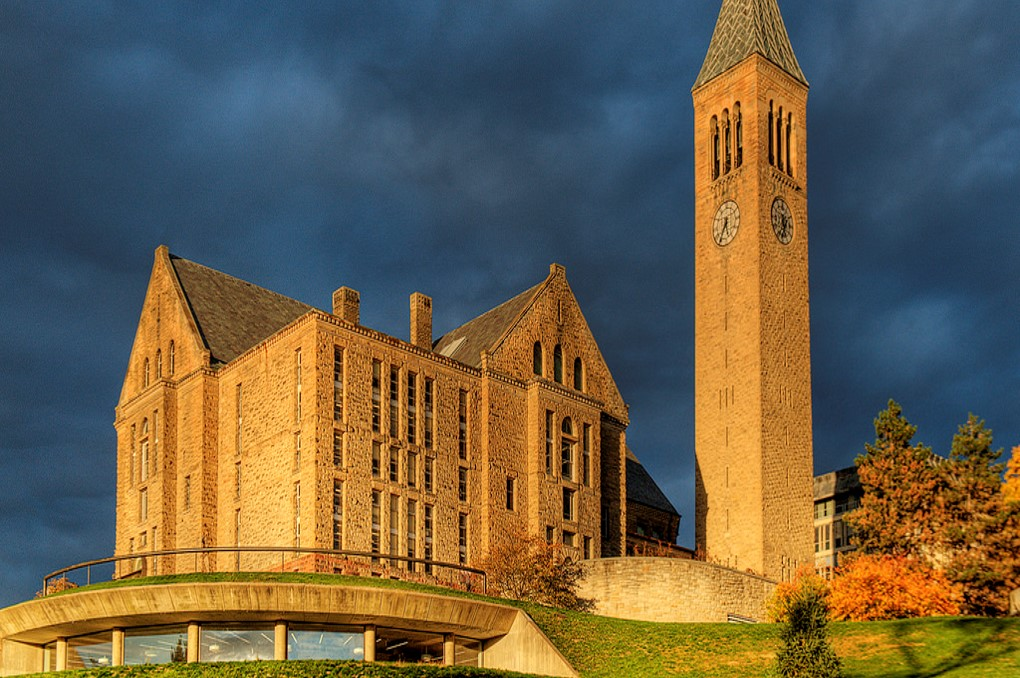# Security Robot

Cornell ECE5725 Project
Yizhou Sun, Yunjie Li

## Introduction

We’ve designed a security robot based on Raspberry Pi which can navigate itself in your home avoiding obstacles and also detect suspicious events (motion) when you leave your house. Once a suspicious event is detected, the robot will send an email to the host which includes a photo to notify that.
In this report we will introduce our design process in details. The security robot mainly consists of two parts: Motion Detection and Navigation. In Motion Detection, a Pi-Camera and the OpenCV module are used to detect moving objects. In the Navigation, we used three ultrasound distance sensors in front, right, left side of the robot to detect the distance to obstacles and the Raspberry Pi (R-Pi) will analyze the and decide what to do in next step.

## Objective

• Build a robot that can navigate in a house and be able to avoid encountered obstacles.
• Detect suspicious events using Pi-Camera and OpenCV.
• Send an email with photo of suspicious event to the host.

## Design & Testing

### Overview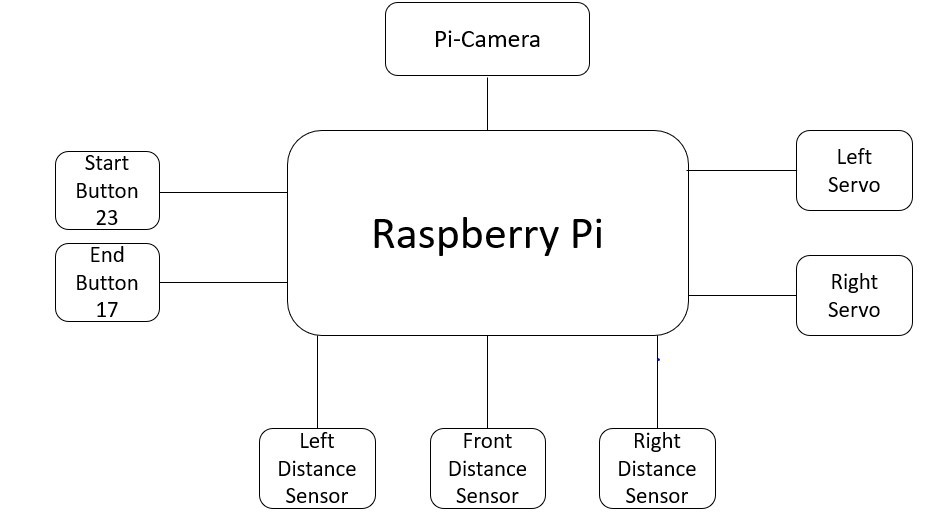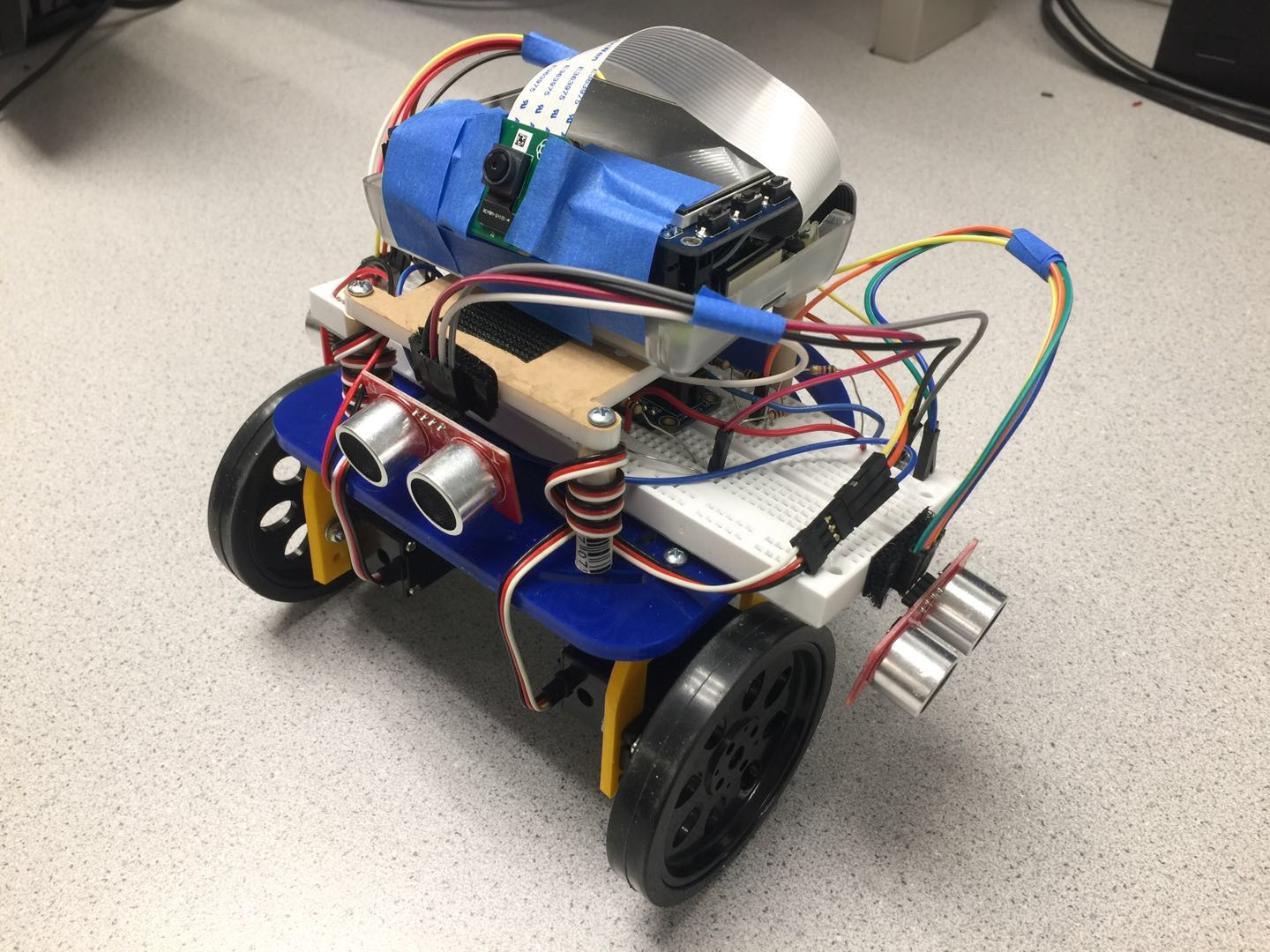Figure 1. System overview and photo of the Security Robot

### Robot Assembly

Camera
We used Raspberry Pi Camera Module V2 as the detection device. Following the instruction , we first connected the camera to Pi via the camera port. Then we started the Pi and and config the system by opening the Raspberry Pi Configuration Tool and setting the camera interface to be enabled. After that we reboot system. And we wrote a simple Python program to test that it works and can caputure video successfully.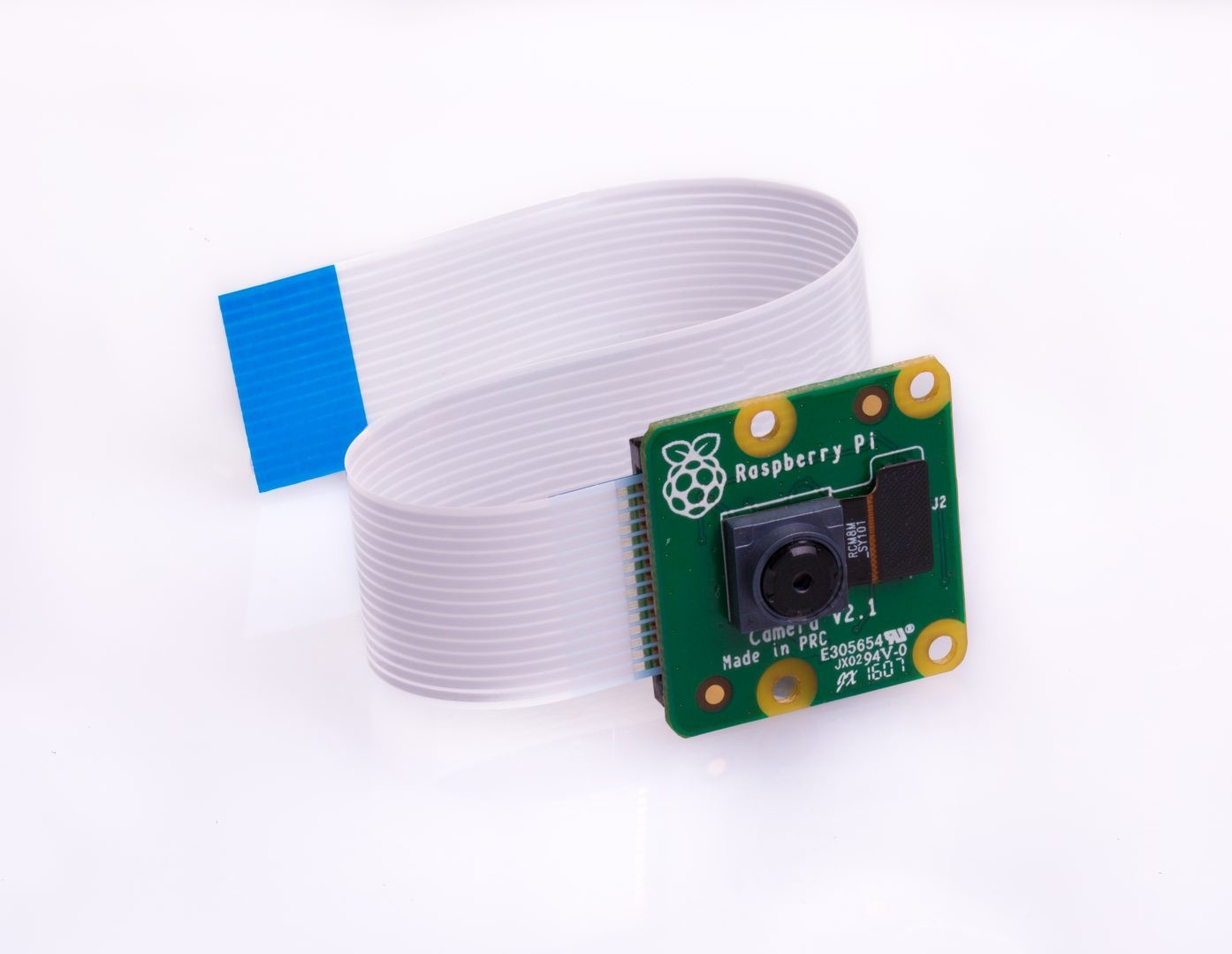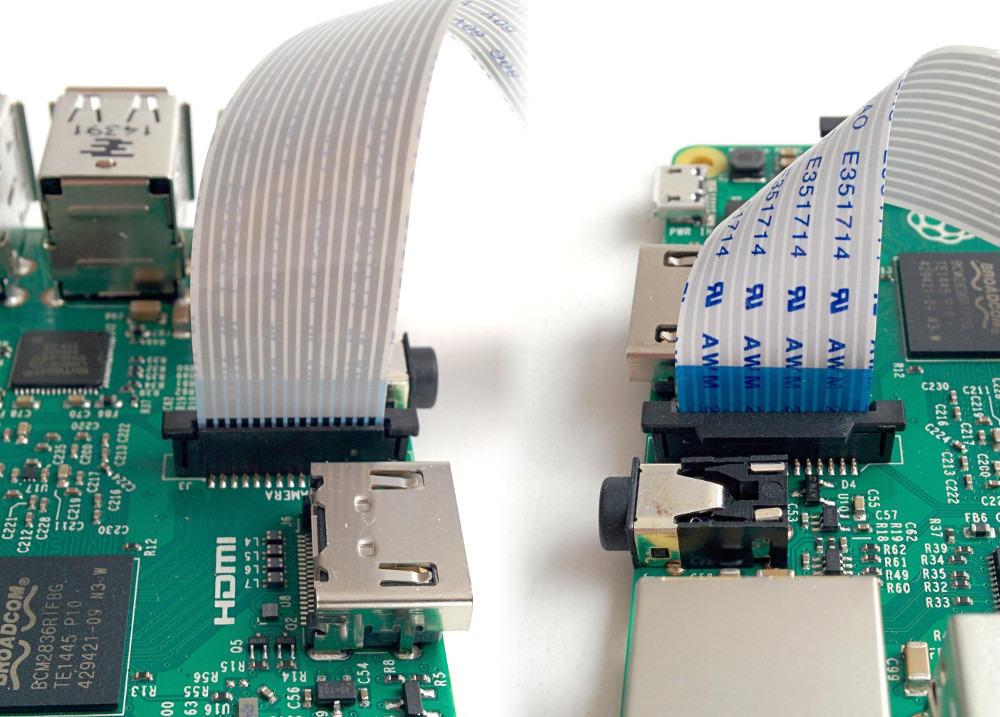Figure 2. Pi-Camera and connection 

Servo Motor
The wheels of our robot were implemented by two Parallax continuous rotation servo motors. Figure 3 is the circuit of the servo. We used pin GPIO 6 and 26 as the outputs pins for two servos and the GPIO python library to generate PWM input signal to control them.
The direction and speed of the servo is determined by the duty cycle of the output signal. After carefully calibration, we set the frequency of the signal to 46.51Hz, to control the robot more easily we used a low speed for the servo motor. The duty cycle for clockwise direction is 6.71%, the duty cycle of counterclockwise is 7.17%.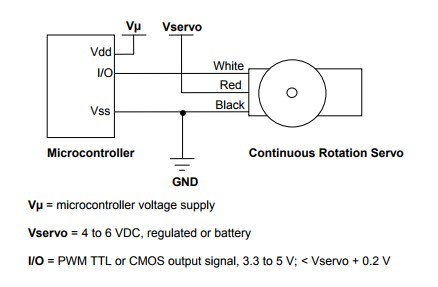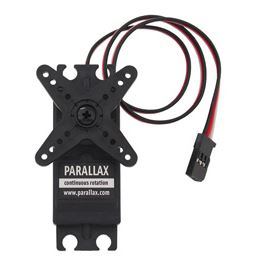Figure 3. Circuit of the servo and its sample image 

Ultrasonic Distance Sensors
To detect the distance between the robot to obstacles, we used three HC-SR04 ultrasonic distance sensors, one was placed at the front, and two at left and right side of the robot respectively.Each distance sensor has four pins, one is connected to 5V power supply and one is connected to ground. The other two pins, ECHO and TRIG, are used to send and receive ultrasonic pulse.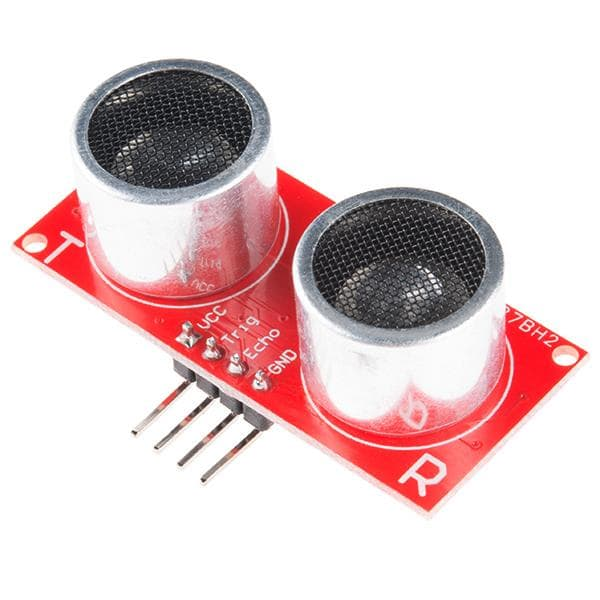Figure 4. Ultrasonic Distance Sensors HC-SR04

The voltage generated by pin ECHO is 5V, but the input pin of Raspberry Pi can only accept 3.3V signal. Therefore, it is necessary to use a voltage divider to bring down the voltage to 3.3V. The final connection can be found in System Design & Schematic. Let t denote the time interval between the time when a pulse is generated pin TRIG and the time when the pulse is received by pin ECHO. Because the speed of sound is about 343m/s, and then we can calculate the distance using the following equation.
Distance = 34300 * t / 2 (cm) = 17150 * t (cm)
For the distance sensor at the front, it connected ECHO to GPIO 18 and TRIG to GPIO 24; For the distance sensor at left, it connected ECHO to GPIO 17 and TRIG to GPIO 22 and for the distance sensor at right, it connected ECHO to GPIO 13 and TRIG ti GPIO 22. While testing the distance sensor, we found that we need to give a small period of time before testing the distance. For example if we test the distance to the front obstacle immediately after the testing the distance to the left, the result would be inaccurate, because the ultrasonic pulse generated by left sensor will affect the sensor at the front, which will give us a wrong answer.

### System Design & Schematic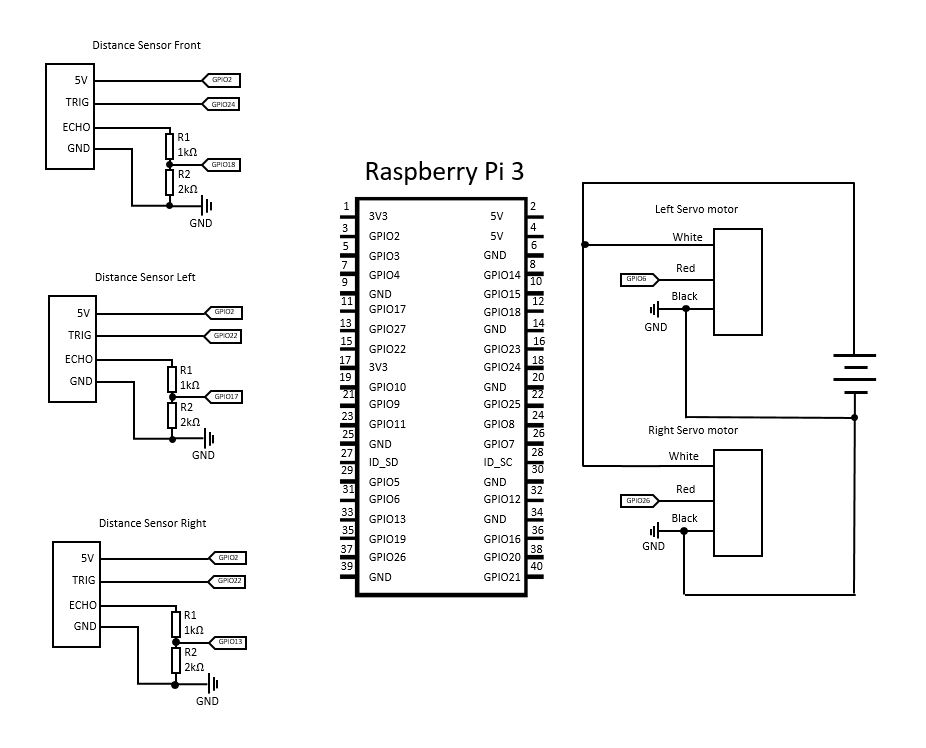### Software Design

Motion Detection
We used Python OpenCV library to implemented the motion detection function. To be able to detect moving object the robot need to be stationary, then we use the Pi-Camera to capture the video and process it. First step is to install OpenCV using following command:
``` sudo apt-get update sudo apt-get install libopencv-dev python-opencv ``` However, we met one issue when installing OpenCV, when we ran command "sudo apt-get install libopencv-dev python-opencv", the terminal shows an error that "The value "stable" is for APT::Default-Release is invalid". But this problem is easy to, we can edit the configuration file “/etc/apt/apt.conf/10defaultRelease” by replacing the word “stable” by “oldstable”. Then ran command “sudo apt-get install libopencv-dev python-opencv”, the OpenCV would be installed successfully.
Next step is to use OpenCV to implement motion detection module. We used the picamera.capture_continuous to capture the image frames from the camera. Then for those frames, we need to do some preprocessing about the frames before detecting the moving objects. We first converte them into grayscale frames, and apply a Gaussian filter to remove the high frequency noise. This can make the detection more precise.
For the first frame we save it as the base frame, then all the next frames i, we will compute a new frame n by the differences between base and frame i. We threshold the new frame n to keep the pixels that have large changes. Then we apply a morphological filter to fill the hole on the new frame. After that we will count the area of the detected object, once the it is larger than the defined minimum area, we view it as a detected object, else we view it as noise. In the end we will add frame i to base frame as a new base frame for next calculation. 
In order to increase the accuracy, only detect moving object in three continuous frames, we view it as a suspicious event, then we mark the object with a green square, save it and send it in email to the host. We tested this algorithm with different parameters to find the best threshold value that has a proper sensitivity that can detect moving object without report the noise of the environment. Following is the sample result generated by our robot, the moving person is marked by a green square.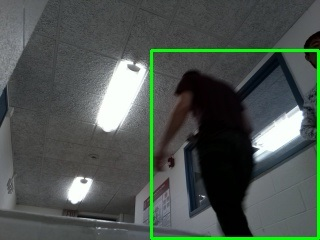Figure 5. Sample result of the motion detection

We've also designed the algorithm to navigate the robot inside a house. The basic idea is to let the robot always move along the left wall. Since this robot is designed to move inside a house, we can always place the robot's left side close to a wall before starting it. And moving along the left wall guarantees that the robot can go through all rooms in most cases.
The first step is the measurement, we measured the exact speed of our robot when moving forward, and the exact angular velocity when it rotates clockwise and counter-clockwise under the duty cycles defined above. We found that its speed is 15 cm/s when moves forward and backward and the angular velocity is 86.12 degree/sec when rotating clockwise and 76.92 degree/sec when rotating counter-clockwise.
In order to do implement the 'left wall' navigation , we've written several functions in python, moveForward(), moveBackward(), turnLeft(), turnRight(), stop() and getDistance(), detail can be seen in code appendix. These functions would change the duty cycle of GPIO output signal to control the servos.
We used a while true loop to control the movement of the robot in a house. At the beginning of each iteration, we call moveForward() and then call time.sleep(1), which let the robot go straight for about 15 cm. Then we call getDistance() to get the distance of the robot to left wall, right wall and front wall. If the distance to 3 wall are all very small, it means a dead end in front, we call function turnRight() and time.sleep(2.09) to let the robot turn 180 degree. Else if the distance to front wall is less than frontLowerBound, we call turnRight() and time.sleep(1.045) to rotate 90 degrees clockwise (turn right) and then move forward as shown in figure below.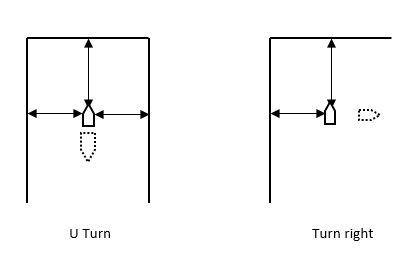Figure 6. Navigation algorithm, U turn and turn right

If it is non of the situation above, it will check leftDist (distance to left wall), if leftDist is greater than leftLowerBound and less than leftHigherBound, we know the robot is in a good situation and continue to move forward. If leftDist is smaller than leftLowerBound, which means that the robot is too close to the left wall, we'll call turnRight(), time.sleep(1), moveForward(), time.sleep(1), turnLeft(), time.sleep(1) continuely to control the robot move apart from the left wall. If leftDist is greater than leftHigherBound but less than leftThreshSpace, which means that the robot is far from the left wall, we call these functions in similar way to make the robot close to the left wall. And if leftDist is greater than leftThreshSpace, which means that there may be a 'door' at the left side, we will make the robot turn left.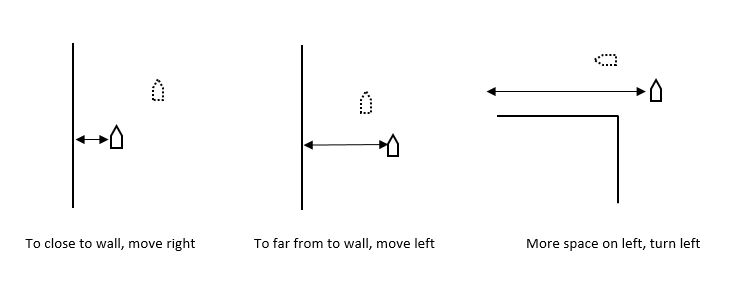After we implemented the first version discussed above, we started to test it. However, we found a problem during the test process: the above algorithm works only when the robot moves strictly straight after the moveforward() is called. But as we can’t control servos very precisely, and the friction on different areas of the ground is not the same, it’s really hard to make the robot move strictly straight.
Then we wrote some new codes to fix the problem. In the beginning of each movement, we first record the oldLeftDist. After the robot moves forward for about l = 15 cm, we check if oldLeftDist is as same as leftDist. If abs(oldLeftDist - leftDist) is greater than some threshold, we rotate the robot for arcsin((oldLeftDist - leftDist)/l) degrees to make sure the robot moves straight. As shown in figure
Also, we added simple recovery function to our navigation algorithm. The recovery part is made to handle the possible collision to wall. In each iteration, the robot will check if frontDist is too smaller, as it already touched the wall, the robot will move backwards, then turn right and move forward.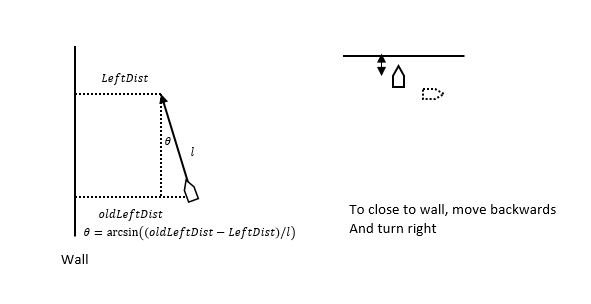## Result & Future Work

We’ve successfully build the security robot as expected. First we assembled the robot according using two servo motors, one Pi-Camera and three ultrasound distance sensors. The servos at left and right side are used to control 2 wheels control motion of the robot. Pi-Camera is used to detect suspicious events is mounted at the top of the robot. Three ultrasound distance sensors are mounted at the front, left and right side. These sensors give distance information to the Raspberry-Pi which helps navigation.

The second part is the software part which consists of motion detection and navigation. In motion detection part, we installed OpenCV library and used it to implemented a motion detection algorithm, and it will save a picture of the detected object and send a email to the host. Navigation part implemented by a ‘along left wall’ algorithm using the distance feedback from threee distance sensors. Moving along the left wall guarantees that our robot can go through all rooms in the house in most cases. The robot can also navigate itself to avoid obstacles and recover from collision to walls. We’ve also designed a function to help the robot move strictly straight.

During the whole project, we faced some issues. The first issue is the OpenCV installation issue, we solved it by changing the variable in the version configuration file. Second issue is the distance sensor issue, we solved it by writing our own function to calculate distance instead of using third-party library. Third issue is that the robot doesn't not always move strictly straight, which makes the movement control difficult, we solved it by carefully calculate the angle between the wall, and adjust the direction.

In the future, we would like to implement a mapping function to save the path. Therefore, after the first traversal of your home, the robot can go much faster in the next traversals because we don’t need to detect the distance any more. We can improve the motion detect to write a more complicated algorithm so that we can detect the moving object while moving. And we can add a microphone on the robot to detect the sound of footsteps or other large noise in the house and we want it to generate alert sound when something is detected.

## Contact

Yizhou Sun (ys775@cornell.edu) Yunjie Li (yl2684@cornell.edu)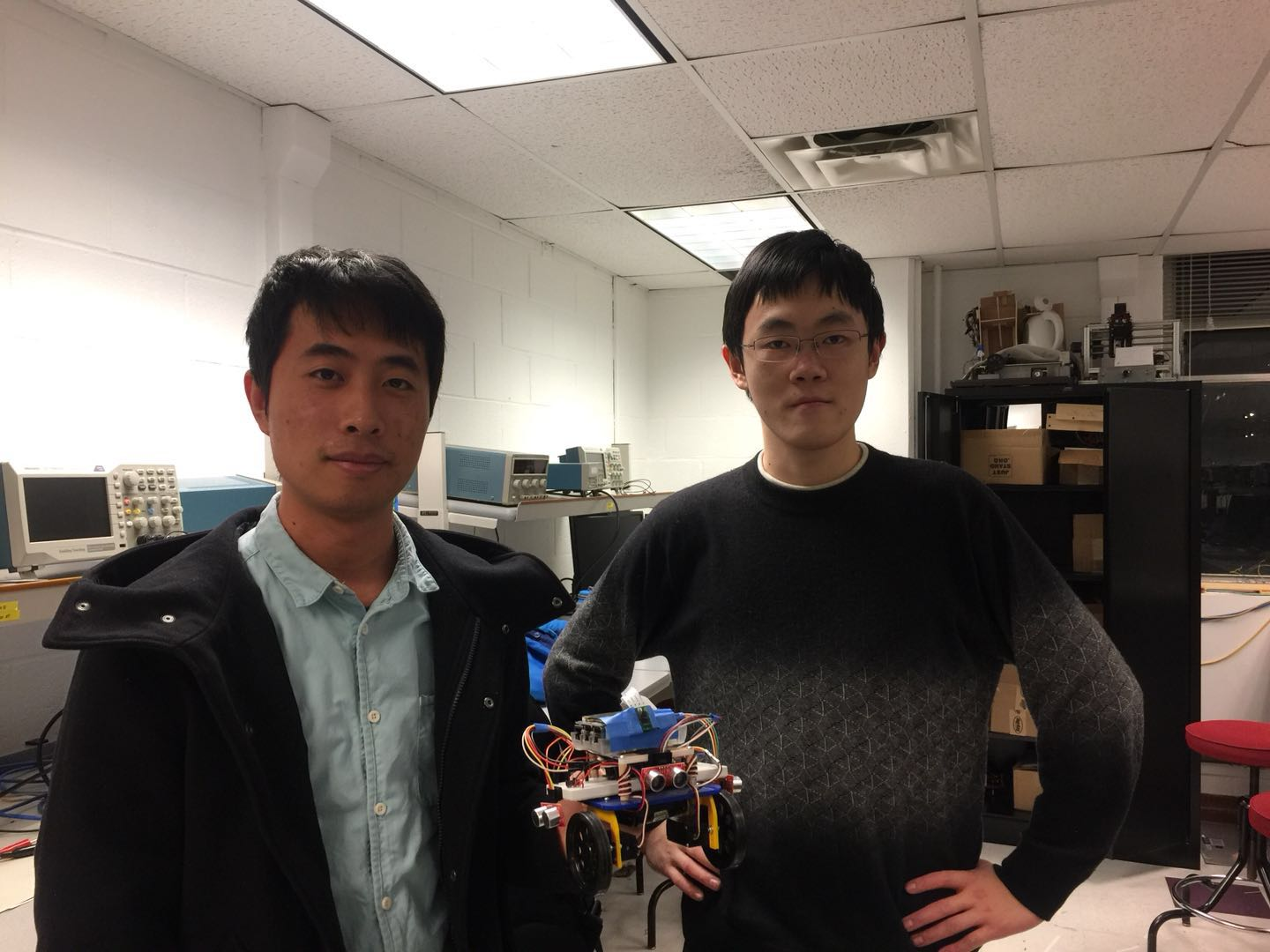## Parts List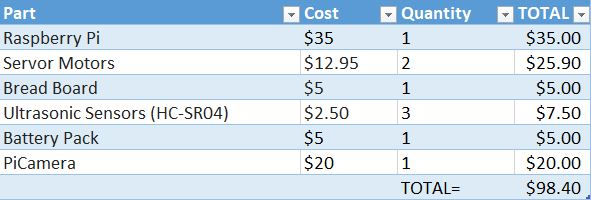## Code Appendix

MyCamera.py
```# Security Robot ECE 5725 Project
# Yizhou Sun, Yunjie Li
# 12-07-2017
# ref:  https://www.pyimagesearch.com/2015/06/01/home-surveillance-and-motion-detection-with-the-raspberry-pi-python-and-opencv/

import cv2
import datetime
import imutils
import json
import time
import sys
import smtplib
import multiprocessing as mp
from picamera.array import PiRGBArray
from picamera import PiCamera
from email.mime.text import MIMEText
from email.mime.image import MIMEImage
from email.mime.multipart import MIMEMultipart

class MyCamera(object):
# initialize picamera
def __init__(self, jsonFile):
print "Initialize PiCamera"
self.camera = PiCamera()
self.camera.resolution = tuple(self.config["resolution"])
self.camera.framerate = self.config["fps"]
self.duration = self.config["duration"]

def detectMotion(self):
print "Start detection"
timeStamp = time.time()
baseFrame = None
numMoved = 0
rawCapture = PiRGBArray(self.camera, size=tuple(self.config["resolution"]))
# capture the frames and process
for frame in self.camera.capture_continuous(rawCapture, format="bgr", use_video_port=True):
frameArr = frame.array
# get grayscale frame and remove noise using GaussianBlur
grayFrame = cv2.GaussianBlur(cv2.cvtColor(frameArr, cv2.COLOR_BGR2GRAY), (17,17), 0)
status = False
if baseFrame is None:
# initialize baseframe
baseFrame = grayFrame.copy().astype("float")
rawCapture.truncate(0)
else:
cv2.accumulateWeighted(grayFrame, baseFrame, 0.5)                   # weighted sum of frames
diffFrame = cv2.absdiff(grayFrame, cv2.convertScaleAbs(baseFrame))  # compute the differences
threFrame = cv2.threshold(diffFrame, self.config["delta_thresh"], 255, cv2.THRESH_BINARY) # threshold the frame
threFrame = cv2.dilate(threFrame, None, iterations=2)               # fill the hole
cnts = cv2.findContours(threFrame, cv2.RETR_EXTERNAL, cv2.CHAIN_APPROX_SIMPLE) # find all contours
cnts = cnts if imutils.is_cv2() else cnts

for contour in cnts:
# threshold small areas and mark it on image
if cv2.contourArea(contour) > self.config["min_area"]:
(x, y, w, h) = cv2.boundingRect(contour)
cv2.rectangle(frameArr, (x, y), (x + w, y + h), (0, 255, 0), 2)
status = True

# if detected some motions save image and return true
if status:
numMoved += 1
if numMoved > self.config["min_moved_frames"]:
cv2.imwrite("warning.jpg", frameArr)
return status
else:
numMoved = 0
# check if run enough time return
if time.time() - timeStamp > self.duration:
return status

if self.config["show_video"]:
cv2.imshow("Video", frameArr)
key = cv2.waitKey(1) & 0xFF
if key == ord("q"):
return status

# clear current frame
rawCapture.truncate(0)

# function that send a warning email to user
def sendEmail():
imgName = "warning.jpg"
msg = MIMEMultipart()
msg['Subject'] = "WARNING: Detected Motions in Your House"

msg.preamble = 'Multipart massage.\n'

text = MIMEText("Hi, please find the attached file")
msg.attach(text)
msg.attach(image)

server = smtplib.SMTP("smtp.gmail.com:587")
server.ehlo()
server.starttls()
server.sendmail(msg['From'], msg['To'], msg.as_string())
server.quit()

if __name__ == '__main__':
if len(sys.argv) < 2:
print "No JSON Config File"
exit(0)

camera = MyCamera(sys.argv)
if camera.detectMotion():
print "detected"
sendEmail()
else:
```
MyRobot.py
```# Security Robot ECE 5725 Project
# Yizhou Sun, Yunjie Li
# 12-07-2017

import RPi.GPIO as GPIO
import time

class MyRobot(object):
def __init__(self):
# setup GPIO and and servo
self.highVal = 7.17
self.lowVal = 6.71
self.leftDc = 0
self.rightDc = 0
self.leftECHO = 17
self.leftTRIG = 22
self.rightECHO = 13
self.rightTRIG = 22
self.frontECHO = 18
self.frontTRIG = 24
GPIO.setmode(GPIO.BCM)
GPIO.setup(6, GPIO.OUT)
GPIO.setup(26, GPIO.OUT)
self.rightServo = GPIO.PWM(6, 46.51)
self.leftServo = GPIO.PWM(26, 46.51)
# init distance sensor
GPIO.setup(self.leftECHO, GPIO.IN)
GPIO.setup(self.leftTRIG, GPIO.OUT)
GPIO.setup(self.rightECHO, GPIO.IN)
GPIO.setup(self.rightTRIG, GPIO.OUT)
GPIO.setup(self.frontECHO, GPIO.IN)
GPIO.setup(self.frontTRIG, GPIO.OUT)
time.sleep(2)
pass

# start the robot
def start(self):
self.rightDc = 0
self.leftDc = 0
self.leftServo.start(self.leftDc)
self.rightServo.start(self.rightDc)
pass

# make robot move forward
def moveForwards(self):
self.rightDc = self.highVal
self.leftDc = self.lowVal
self.leftServo.ChangeDutyCycle(self.leftDc)
self.rightServo.ChangeDutyCycle(self.rightDc)
pass

# make robot stop
def stop(self):
self.rightDc = 0
self.leftDc = 0
self.leftServo.ChangeDutyCycle(self.leftDc)
self.rightServo.ChangeDutyCycle(self.rightDc)
pass

# make robot turn right
def turnRight(self):
self.rightDc = self.highVal
self.leftDc = self.highVal
self.leftServo.ChangeDutyCycle(self.leftDc)
self.rightServo.ChangeDutyCycle(self.rightDc)
pass

# make robot turn left
def turnLeft(self):
self.rightDc = self.lowVal
self.leftDc = self.lowVal
self.leftServo.ChangeDutyCycle(self.leftDc)
self.rightServo.ChangeDutyCycle(self.rightDc)
pass

# make robot move backward
def moveBackwards(self):
self.rightDc = self.lowVal
self.leftDc = self.highVal
self.leftServo.ChangeDutyCycle(self.leftDc)
self.rightServo.ChangeDutyCycle(self.rightDc)
pass

# get distance to left
def getDistLeft(self):
t = GPIO.PWM(self.leftTRIG, 10)
t.start(0.1)

while GPIO.input(self.leftECHO)==0:
pulse_start = time.time()
while GPIO.input(self.leftECHO)==1:
pulse_end = time.time()

pulse_duration = pulse_end - pulse_start
distance = pulse_duration * 17241
t.stop()

return distance

# get distance to right
def getDistRight(self):
t = GPIO.PWM(self.rightTRIG, 10)
t.start(0.1)

while GPIO.input(self.rightECHO)==0:
pulse_start = time.time()
while GPIO.input(self.rightECHO)==1:
pulse_end = time.time()

pulse_duration = pulse_end - pulse_start
distance = pulse_duration * 17241
t.stop()

return distance

# get distance to front
def getDistFront(self):
t = GPIO.PWM(self.frontTRIG, 10)
t.start(0.1)

while GPIO.input(self.frontECHO)==0:
pulse_start = time.time()
while GPIO.input(self.frontECHO)==1:
pulse_end = time.time()

pulse_duration = pulse_end - pulse_start
distance = pulse_duration * 17241
t.stop()

return distance

# quit robot
def quit(self):
GPIO.cleanup()
pass
```
mainFunction.py
```# Security Robot ECE 5725 Project
# Yizhou Sun, Yunjie Li
# 12-07-2017

import os
import time
import RPi.GPIO as GPIO
import pygame
from MyRobot import MyRobot
import datetime
from pygame.locals import *
import MyCamera
import math

# global variables
size = width, height = 320, 240
white = 255, 255, 255
black = 0, 0, 0
FrontLowThreshold = 30
LeftLowThreshold = 11
LeftHighThreshold = 18
RightLowThreshold = 10
LeftThrehSpace = 50

# function that use pygame to draw screen and wait for start button is pressed
def startUI(screen):
screen.fill(black) # erase screen
GPIO.setmode(GPIO.BCM)
GPIO.setup(27, GPIO.IN, pull_up_down=GPIO.PUD_UP)
GPIO.setmode(GPIO.BCM)
GPIO.setup(23, GPIO.IN, pull_up_down=GPIO.PUD_UP)
my_font = pygame.font.Font(None, 20)
text = my_font.render('Press button 4, 3 to start, end', True, white)
rect = text.get_rect(center = (160, 120))
screen.blit(text, rect)
pygame.display.flip()
waitForStart()

# callback function to quit
def Gpio23_callback(channel):
GPIO.cleanup()
exit(0)

def waitForStart():
# detect touch events
while True:
time.sleep(0.2)
if (not GPIO.input(23)): # button 27 has been pressed
return

# do detection for two directions and write to a log
def doDetection(camera):
i = 0
logFile = open("log.txt","a")
curTime = datetime.datetime.now()
logFile.write("\n\n")
logFile.write(curTime.strftime("%Y-%m-%d %H:%M"))
logFile.write(": Start to detect motion")

while i < 2:
myRobot.turnRight()
time.sleep(1)
myRobot.stop()
i += 1
if camera.detectMotion():
logFile.write("\nDetected object, send email !!")
print "Detected object, send email !!"
MyCamera.sendEmail()
else:
logFile.write("\nNothing found")
print "Nothing found"

myRobot.turnLeft()
time.sleep(2)
logFile.close()

if __name__ == "__main__":
# enviroment variables
os.putenv('SDL_VIDEODRIVER', 'fbcon') # Display on piTFT
os.putenv('SDL_FBDEV', '/dev/fb1')
os.putenv('SDL_MOUSEDRV', 'TSLIB')    # Track mouse clicks on piTFT
os.putenv('SDL_MOUSEDEV', '/dev/input/touchscreen')
logFile = open("log.txt","w")
curTime = datetime.datetime.now()
logFile.write("\n\n")
logFile.write(curTime.strftime("%Y-%m-%d %H:%M"))
logFile.write(": Start myRobot and initialize")
pygame.init()
pygame.mouse.set_visible(False)
screen = pygame.display.set_mode(size)

startUI(screen)
# initial MyRobot
myRobot = MyRobot()
myRobot.start()
# get configuration file
camera = MyCamera.MyCamera("/home/pi/final_project/conf.json")

# left 230 degree 3s 90-1.17 30-0.39
# right 260 degree 3s 90-1.045 30-0.348
i = 1
logFile.write("\nStart moving")
logFile.close()
oldLeft = left = 0
while True:
oldLeft = myRobot.getDistLeft()
myRobot.moveForwards()
time.sleep(1.5) # forward 5cm
myRobot.stop()

left = myRobot.getDistLeft()
time.sleep(0.5)
right = myRobot.getDistRight()
time.sleep(0.5)
front = myRobot.getDistFront()
print "Left", round(left,2), "cm", "Right", round(right,2), "cm", "Front", round(front,2), "cm"

if abs(oldLeft - left) > 3 and abs(oldLeft - left) < 10:
degree = math.asin(abs(oldLeft - left)/13.0) * 180 / 3.1415
if (oldLeft > left):
myRobot.turnRight()
time.sleep(degree * 1.045 / 90)
myRobot.stop()
else:
myRobot.turnLeft()
time.sleep(degree * 1.17 / 90)
myRobot.stop()

if i == 10:
# detection
doDetection(camera)
i = 1
else:
i += 1

if front <= 10:
print "move back"
myRobot.moveBackwards()
time.sleep(1)
myRobot.stop()
myRobot.turnRight()
time.sleep(1.045)
myRobot.stop()

elif right <= RightLowThreshold and left <= LeftLowThreshold and front <= FrontLowThreshold:
print "U turn"
myRobot.turnLeft()
time.sleep(2.09)
myRobot.stop()

elif left > LeftThrehSpace:
print "turn left (door?)"
myRobot.turnLeft()
time.sleep(1.17)
myRobot.moveForwards()
time.sleep(3)
myRobot.stop()

elif front <= FrontLowThreshold:
print "turn right"
myRobot.turnRight()
time.sleep(1.045)
myRobot.stop()

elif left > LeftHighThreshold:
print "turn left to close the wall"
myRobot.turnLeft()
time.sleep(0.39)
myRobot.stop()
myRobot.moveForwards()
time.sleep(1)
myRobot.stop()
myRobot.turnRight()
time.sleep(0.348)
myRobot.stop()

elif left < LeftLowThreshold:
print "turn right to leave the wall"
myRobot.turnRight()
time.sleep(0.348)
myRobot.stop()
myRobot.moveForwards()
time.sleep(1)
myRobot.stop()
myRobot.turnLeft()
time.sleep(0.39)
myRobot.stop()
```